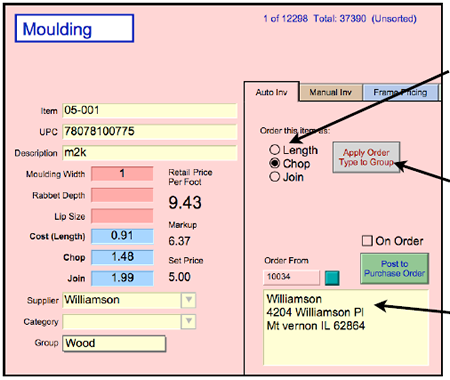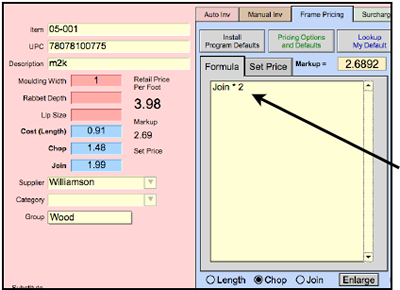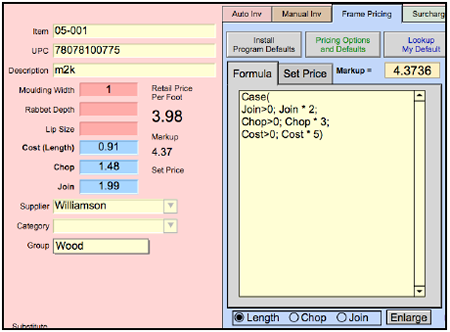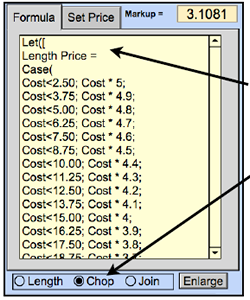### How to Price by Join / Order by Chop or Length

FrameReady formulas offer the flexibility to price moulding by one method but order your moulding by another. E.g. Price by “Join times two” but order by “Length”. The advantage of this method is that the retail price per foot will always remain the same, regardless of how you order the moulding.Your price is consistent. Even if you change it on a work order.

#### Here’s how it works:

1. In the Price Codes file, the radio button will identify how each moulding will be ordered. Mark the circle to identify how you will order the moulding.

You can use the Apply Order Type to Group button to mark the circle for all Moulding records or individual groups (E.g. Supplier: Roma and Group: Wood)

Which company you order the moulding from is located below the radio buttons.

Now comes the deciding factor… Your Pricing Formula.There are a few different ways to set up your pricing formula. The formula in this example is very simple with no other pricing options.

#### Option #1

Join * 2 which means that only the Join price is used. Even though the radio button is set to order by Chop, FrameReady will price based on the Join cost field only.

Join 1.99 x Markup 2 = 3.98 Retail Price per Foot.

So why does the Markup = 2.6892 appear in the box above the formula? FrameReady knows that I am ordering this as a Chop, and, in order to have the retail price equal 3.98, it calculates that (Chop) 1.48 * 2.6892 is the same as (Join) 1.99 * 2. Both create a Retail Price Per Foot of 3.98. This number is just an FYI and does not require any work on your part.

However, the above formula will only work if the supplier has provided a Join wholesale cost. If the supplier has not provided a wholesale cost for Join, your retail price will be zero. This formula does not leave any margin for error.

#### Option #2In the example below, you will see how we have set up the pricing to accommodate all variables. Because Join is the first option listed, it is given top priority. However, because we have added “Join>0” this means that there must be a quantity greater than zero in the blue Join wholesale cost field.

If the Join cost field is empty, the program looks to the Chop wholesale cost. If it also is empty, then the Length cost is used.

In this example, we are ordering by Length. Notice how the Markup = 4.3736. This is the number necessary to have the retail price equal 3.98 (which is the amount calculated by Join * 2).

NOTE:If you are using the FrameReady Default pricing formula (shown below), which starts with:

Let([

Length Price =

then, your pricing is determined by the radio button (circle) which is marked as the order type (located below the formula window).

If you change the radio button, then the Retail Price per Foot will also change. Therefore, the default formula will only price moulding by your method of order and cannot be used for the pricing option described above this note.